1. Worksheets>
2. Math>
3. Geometry>
4. Parallel, Perpendicular and Intersecting Lines

# Parallel, Perpendicular and Intersecting Lines Worksheets

This module deals with parallel, perpendicular and intersecting lines. Exercises and word problems will help improve the skills to identify and differentiate between parallel, perpendicular and intersecting lines.

Printable charts

Self-descriptive charts will help you learn symbolic representation and characteristics of parallel, perpendicular and intersecting lines.Identify the type

These printable worksheets will assist the students to differentiate the lines based on their geometrical properties. Identify whether the lines are parallel, perpendicular or intersecting lines.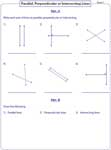Types of lines: MCQ

From the given figure, identify each pair of lines as parallel, perpendicular or intersecting lines. Choose the correct answer from the given options.Pair of lines in geometrical figures

This section is subdivided into two parts.

Part A: Identify the type of lines as indicated by the arrows in each geometrical figure.

Part B: Identify the number of parallel and perpendicular lines from the geometrical figures.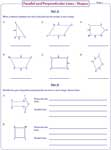Parallel lines and the transversal

From the given sketch, analyze the transversal line. Identify the given lines and answer the questions in the given worksheets.Real-life objects: Parallel vs Perpendicular

Try to identify whether the pair of lines is parallel or perpendicular from the objects you are using in day-to-day life.Parallel and perpendicular streets - Road map

Examine the given road map to identify parallel and perpendicular streets. Answer the questions related to the road map.Prove the relationship: Points and slopes

This section consists of exercises related to slope of the line. Apply slope formula, find whether the lines are parallel or perpendicular. Justify your answer.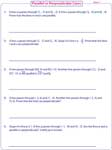Points and slopes: Finding unknown

In these worksheets, the relation between the lines is given. Find the missing coordinate in each problem.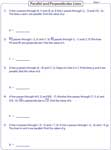Prove the relationship: Equations and slopes

Apply the slope formula for the given equations. Find whether the lines are parallel or perpendicular. Justify your answer.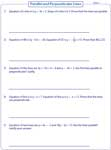Equations and slopes: Finding unknown

In these worksheets, the relation between the lines is given. Find the unknown parameter in the equation.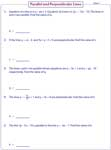Equations, points and slopes

Calculate the slope of the equation. Prove that the lines are parallel or perpendicular. Justify your answer.Stock up your library with the complete set of worksheets on parallel, perpendicular and intersecting lines.

Related Worksheets